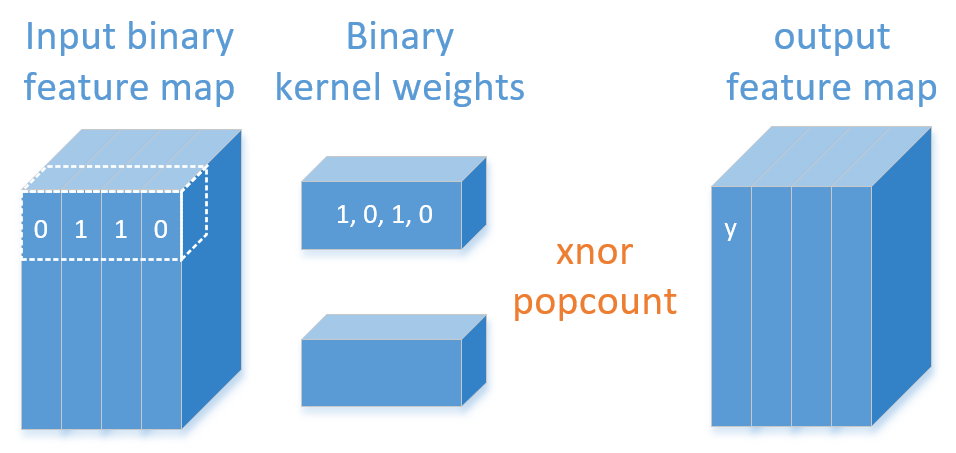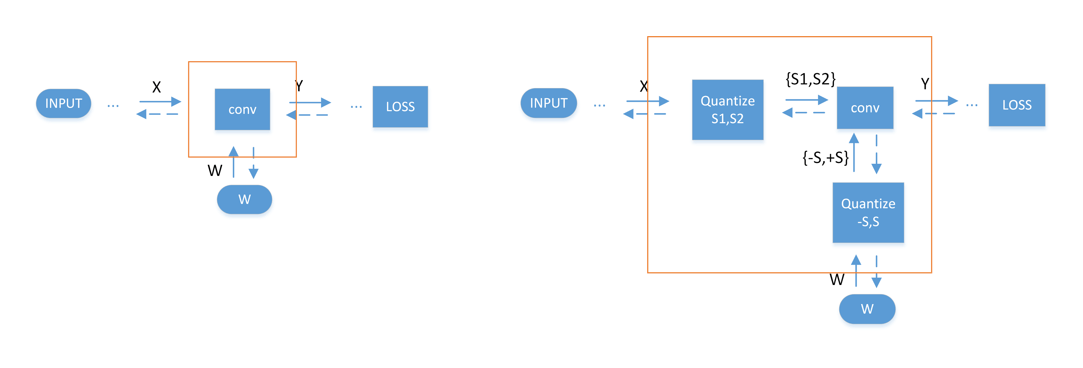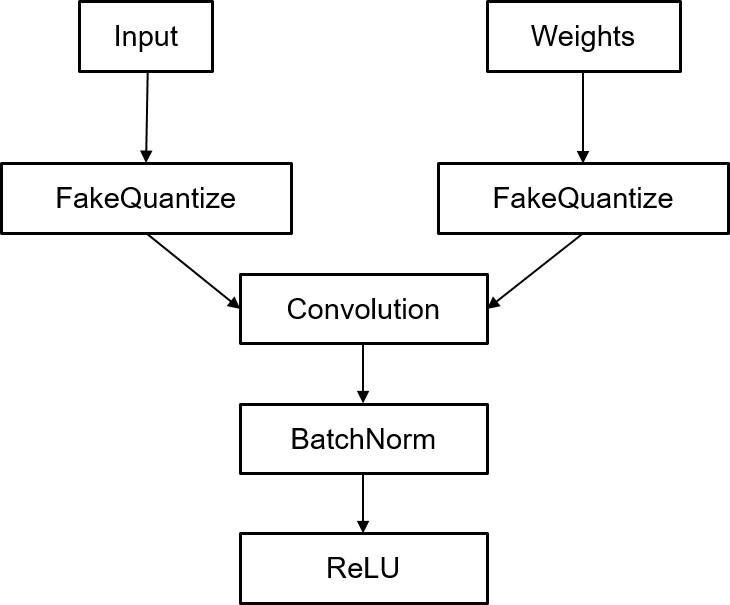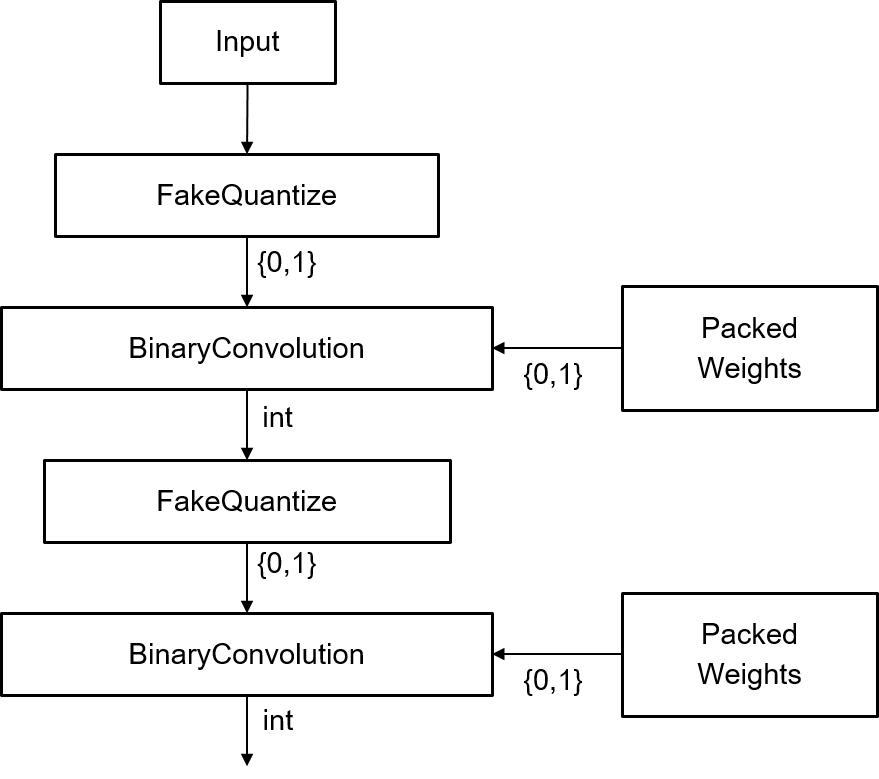# Improving DL Performance Using Binary Convolution Support in OpenVINO Toolkit

Convolutional neural networks (CNNs) are a class of deep neural networks often used to analyze visual imagery. The performance of CNNs is heavily constrained by the performance of the convolution layer on the target platform. Typically, convolution consumes a majority of the network bandwidth — so acceleration of the convolution layer will directly result in acceleration of the entire network.

There is a limit to primitive acceleration imposed by the hardware itself. One way to proceed is to reduce the precision of computations and perform more computations per cycle. Reduced precision computations must be efficiently supported by hardware capabilities – for example, int8 computations only make sense on platforms that fully support it.

Modern CPUs are designed to work efficiently with a few data types: Floating Point 32 (FP32) and integers (int8, int16 and int32). However, binary operations like bitwise-and/or/xor are inherently the most efficient type of operations. Binary operations combine high throughput with low memory pressure and are ideal for lowering precision. One way to accelerate networks using binary primitives is to replace standard convolutions with binary convolutions.

Binary networks show comparably good results in classification and object recognition tasks when compared with full precision networks in terms of quality. Binary convolutions are efficient in terms of memory and computation, while being very accurate in vision workloads running on edge devices with limited memory and computational power resources.

### Neural networks binarization

In binary convolution activations, vector X and weights vector W can take only two values (e.g. 0 or 1). So the multiplication in convolution can be replaced with the bitwise XNOR operation. To make final convolutional summation we can use the “popcount” instruction. Thus, the output value of the convolution can be defined as y =  popcount (W XNOR X).Figure 1. Binary convolution.

During the binarization process, selected convolutional layers of the original CNN are replaced with binary convolution alternatives. When replacing floating point weights with binary, some information is lost. To compensate, an additional fine-tuning of the network in binary format is applied. So there is no calibration-like procedure (as there is for int8 quantization) to get a highly accurate binary net. Moreover, it is not always possible to binarize all layers to get acceptable accuracy level. Often, first and last layers should be kept in a higher precision format like FP32 or int8. Essentially, the resulting model is always run in mixed precision which requires a dynamic switch of the precision in runtime. To do that, the network is modified by inserting a special quantization layer for input activations and weights that converts any full-precision value into two possible values -S or +S (so-called “fake” quantization). Note, to avoid extra calculations these values should be symmetrical in case of weights (-S and +S).

After such binarization-aware training, the OpenVINO™ Model Optimizer tool takes this model and converts the discrete set of floating point values to real binary values by performing a series of linear transformations. The model optimizer is part of the OpenVINO™ Toolkit that enables CNN-based deep learning inference and speeds performance of computer vision applications on a wide range of Intel®-based accelerators — including CPUs, GPUs, VPUs, and FPGAs — using a common API.Figure 2. Left: An ordinary full-precision convolution. Right: Fake quantization (floating point activations and weights are converted into a discrete set of floating point values which are then convolved).

### Train Binary Models Compatible with OpenVINO Toolkit

To provide training capabilities to the OpenVINO community, we are releasing support of binary models in the Neural Network Compression Framework (NNCF) which is a part of OpenVINO Training Extensions. NNCF is built on top of the PyTorch framework and supports a wide range of DL models for various use cases. It also implements quantization-aware training as a mainstream feature for model compression.

NNCF compression procedure relies on the configuration file to provide information about what layers will be “binarized”. However, the process of the layers selection is difficult and requires a deep knowledge of the domain-specific model structure. Otherwise, the final accuracy of the binary model may be not satisfactory.

### Representation of binary models

OpenVINO Model Optimizer accepts a pre-trained binary model in ONNX format. To be able to represent flow with a discrete set of values in a model, we added our own ONNX operator as an extension to the default ONNX operator set. This operator, called FakeQuantize, implements a uniform quantization process in the same way it is implemented in the forward pass in the training. That means that this operator does “fake” quantization by taking floating point values and producing clipped, scaled, shifted and rounded floating point values from a discrete set that is specified by FakeQuantization parameters.

The following pseudo-code shows how the FakeQuantize operator is implemented. It has 5 inputs: a tensor to be quantized, clipping minimum and maximum limits for input values, and minimum and maximum values of an output range that input values should be mapped to. FakeQuantize has one attribute: “levels” that specifies the number of quantization levels in the output range.

```def FakeQuantize(input, input_min, input_max, output_min, output_max, levels): if x <= input_min:       output = output_min elif x > input_max:       output = output_max else:       # input_min < x <= input_max       output = round( (x - input_min) / (input_max - input_min) * (levels-1)) / (levels-1) * (output_max - output_min) + output_min```

Other operators in the model are regular floating point operators from the default ONNX operator set. Using the FakeQuantize operator allows us to easily extract the model from a training framework to the ONNX model without doing “real” quantization beforehand — and without the need to introduce a special version of operations for discrete data processing, like the binary convolution. The real quantization process as well as specialized quantized operations are part of OpenVINO training extensions.Figure 3. Typical fragment of ONNX model with FakeQuantize operators. FakeQuantize takes floats and maps to different and discrete set of floating point values that were deduced during training (usually not 0 and 1 as in “real” binarization).

### Converting the ONNX Model to an OpenVINO Model

The OpenVINO Model Optimizer tool takes the ONNX model with FakeQuantize operators and converts it to a “real”quantized model accepted by the toolkit’s Inference Engine. During conversion, several optimization transformations are applied in order to reduce floating point values used in the source model to values 0 and 1.

For example, as shown in Figure 3, FakeQuantize that processes Weights is transformed into a form where it produces only -1 and +1 as output. To keep the model correct, the corresponding scale from this FakeQuantize is moved through the convolution to the output and kept as a channel-wise multiplication operation. A similar thing happens with FakeQuantize in the Input block; in this case it may be required to pass an additive term through the convolution, depending on the output range of the corresponding FakeQuantize operation.

Then, BatchNorm and all collected addition and multiplication operations after convolution is simplified and united with the next ReLU and FakeQuantize operation before the next convolution (if any).

In the end of the transformation process the regular convolution can be replaced by BinaryConvolution and weights can be represented in a packed format where each element occupies only a single bit, resulting in32x compression ratio. In this case, FakeQuantize operators really do quantization to 0 and 1 values and expressions used in FakeQuantize implementation is simplified.Figure 4. Two consecutive convolutions after the transformation process.

### Pretrained Binary Models in OpenVINO Toolkit

In OpenVINO Toolkit Pre-Trained Models, we delivered four networks with binary convolutions for preview: three object detection networks with a modified version of MobileNet v1 as a backbone: face-detection-adas-binary-0001, pedestrian-detection-adas-binary-0001, vehicle-detection-adas-binary-0001 and one classification network, resnet50-binary-0001. To maintain accuracy, convolutions in some layers were kept in floating point format. For example, in the binary version of ResNet50, the first convolutional layer, last convolutional layer and shortcut layers were kept in floating point format. For the binary version of SSD detectors, eleven 1×1 convolutional layers were trained as binary (this is approximately 80% of all convolutional calculations).

These networks were all tuned with a special quantization layer as described above. The accuracy results of reference floating point nets compared to the same nets with binary convolutions are shown in the table below, in terms of Average Precision for detection nets and  top-1 accuracy on ImageNet for the classification net. Accuracy results were collected using Accuracy Checker tool from Open Model Zoo repository.

Model FP32 Version Binary Version
resnet50 76.15% TOP-1 ACC 70.69% TOP-1 ACC

### Performance

##### CPU

CPUs are inherently efficient in processing binary operations, but our 10th generation Intel® Core™ processor family introduces support for vectorized popcount operation which makes computation of binary convolution even more efficient. Comparison of binary topologies vs. their fp32 counterpart for batch size = 1 is given below.

Configuration: Intel® Core™ i7-8700 Processor @ 3.20GHz with 64 GB RAM, OS: Ubuntu 16.04.6 LTS, Kernel: 4.15.0-29-generic

Model Speedup: binary vs FP32 (latency mode) Speedup: binary vs FP32 (throughput mode)
resnet50 2.3 2.23

Configuration: Intel® Core™ i7-1065G7 CPU @ 1.30GHz with 16 GB RAM, OS: Ubuntu 16.04.6 LTS, Kernel: 4.15.0-54-generic

Model Speedup: binary vs FP32 (latency mode) Speedup: binary vs FP32 (throughput mode)
resnet50 3.53 3.33

##### iGPU

Configuration: Intel® Core™ i7-8700 Processor @ 3.20GHz (Intel® UHD Graphics 630) with 64 GB RAM, OS: Ubuntu 16.04.6 LTS, Kernel: 4.15.0-29-generic, OCL runtime version: 19.04.12237

Model Speedup: binary vs FP16 (latency mode) Speedup: binary vs FP16 (throughput mode)